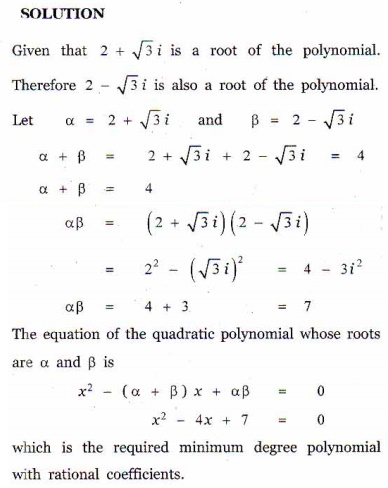Home | | Maths 12th Std | Exercise 3.2: Polynomial Equation in Geometry

# Exercise 3.2: Polynomial Equation in Geometry

Maths Book back answers and solution for Exercise questions - Find a polynomial equation of minimum degree with rational coefficients

EXERCISE 3.2

1. If is real, discuss the nature of the roots of the polynomial equation 2x2 + kx = 0 , in terms of k.2. Find a polynomial equation of minimum degree with rational coefficients, having 2 + √3i as a root.3. Find a polynomial equation of minimum degree with rational coefficients, having 2i + 3 as a root.4. Find a polynomial equation of minimum degree with rational coefficients, having √5 - √3 as a root.5. Prove that a straight line and parabola cannot intersect at more than two points.1. When k < 0 , the polynomial has real roots.

When k = 0 or k = 8 , the roots are real and equal.

When 0 < k < 8 the roots are imaginary.

When k > 8 the roots are real and distinct.

2. x2 - 4x + 7 = 0

3. x2 - 6x +13 = 0

4. x4 -16x2 + 4

Tags : Problem Questions with Answer, Solution , 12th Mathematics : UNIT 3 : Theory of Equations
Study Material, Lecturing Notes, Assignment, Reference, Wiki description explanation, brief detail
12th Mathematics : UNIT 3 : Theory of Equations : Exercise 3.2: Polynomial Equation in Geometry | Problem Questions with Answer, Solution